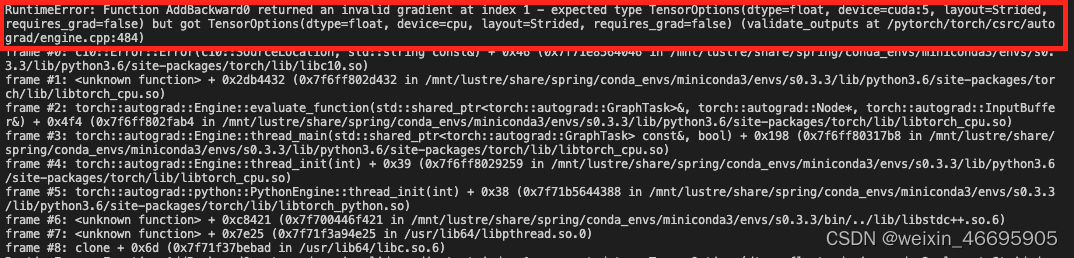# [Solved] pytorch loss.backward() Error: RuntimeError: Function AddBackward0 returned an invalid gradient at index 1…

How to Solve pytorch loss.backward() Error

The specific errors are as follows:In fact, the error message makes it clear that the error is caused by device inconsistency in the backpropagation process.
In the code, we first load all input, target, model, and loss to the GPU via .to(device). But when calculating the loss, we initialize a loss ourselves
loss_1 = torch.tensor(0.0)
This way by default loss_1 is loaded on the CPU.
The final execution loss_seg = loss + loss_1
results in the above error for loss_seg.backward().

Modification method:
Modify
loss_1 = torch.tensor(0.0)
to
loss_1 = torch.tensor(0.0).to(device)
or
loss_1 = torch.tensor(0.0).cuda()